Ratio Worksheets

Ratio worksheets have numerous skills to enhance the numerical ability of children. These worksheets include part-to-part ratios, part-to-whole ratios, equivalent ratio, finding a part of the ratio from a whole number and vice versa. Also learn ratio by drawing and coloring activities.

Ratio Worksheets based on Pictures

Ratio of part-to-part

In these part-to-part ratio worksheets, a set of pictures are shown. Find the part-to-part ratio. In level 1, the pictures are in order and in level 2, the pictures are random.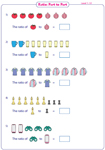Level 1:

Level 2:

Ratio of part-to-whole

Find the part-to-whole ratio from a set of pictures in these worksheets. Write the ratio to compare two quantities and three quantities.Level 1:

Level 2:

Ratio in Three Ways

Ratio in three ways: Pictures

Each ratio worksheet has a set of pictures depicted. Express the proportion of the two quantities in words, ratio and fraction (2 to 3, 2:3, 2/3).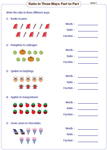Ratio in three ways: Standard

The quantity of the ratio is expressed in words. Read each sentence to express the ratio in three ways and tabulate it. Each worksheet has one word problem for practice.Ratio Activities

Draw the picture

Draw the basic shapes in this activity to show the given ratio in each problem. Circles, squares, rectangles and more shapes are to be drawn.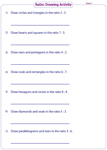Color the shapes

Splendid coloring activity is here for kids to make them understand ratio in a better way. Example: Color 2 umbrellas red and 4 umbrellas green. What is the ratio of red umbrellas to green umbrellas?Reducing Ratio

Reduce to lowest term: No unit conversion

In these worksheets, ratios with real-life units are given. Reduce the ratio to its simplest term. Each sheet has one word problem.Easy:

Moderate:

Reduce to lowest term: Requires Unit Conversion

Reduce the ratio with metric and customary units. Convert the real-life units to a common unit and reduce to its lowest term. One word problem per page.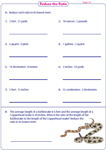Easy:

Moderate:

Dividing Quantities Based on Ratio

Finding a part from a whole

Divide the quantity to find the share of each part from the given ratio and the whole number. Word problems included.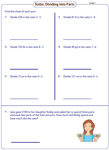Finding a whole from a part

The ratio and the value of one part are given. Find the share of another part and also the total. These worksheets also have word problems for practice.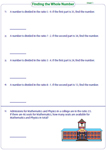Divide into three parts

For the given ratio, divide the quantity into three parts. One word problem per page on sharing money is given in this ratio worksheet.Ratio Word Problems

A huge collection of ratio word problems based on finding the ratio between two quantities, reducing the ratio, part-to-part, part-to-whole and more. Read the graph and answer the word problems that follow.Ratio Word Problem Worksheets(27 Worksheets)

Equivalent Ratio

Writing equivalent ratio

Write any two equivalent ratios for the given ratio in section A. Complete the equivalent ratio table in section B.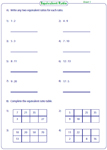Checking Equivalency

In section A, check the equivalency of ratios. In section B, determine the unknown part of the ratio. (Ex: 2/3 = k/6). Word problems are included.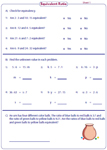Proportions Worksheets

This section covers a number of topics like identify the proportion, form a proportion, constant of proportionality, unit rate, solving proportion, word problems and more.Proportions Worksheets (120 worksheets)

The entire collection of worksheets on ratios can be downloaded in a jiffy!

Related Worksheets